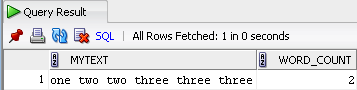As of Oracle 11 you can use the REGEXP_COUNT function to count the number of occurrences of a word or string in a varchar. However, if you’re using Oracle 10 you may be able to achieve something similar as shown below.

```select mytext,
nvl(length(regexp_replace(regexp_replace(replace(mytext, '~', ' '),
'two', '~', 1, 0, 'i'), '[^~]', '')), 0) as word_count
from (select 'one two two three three three' as mytext
from dual);```

In this example we count how many times the word ‘two’ appears in the text: ‘one two two three three three’.